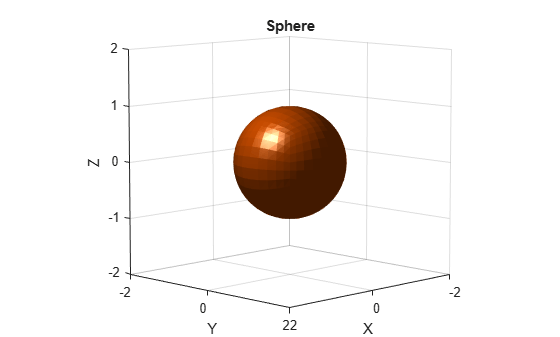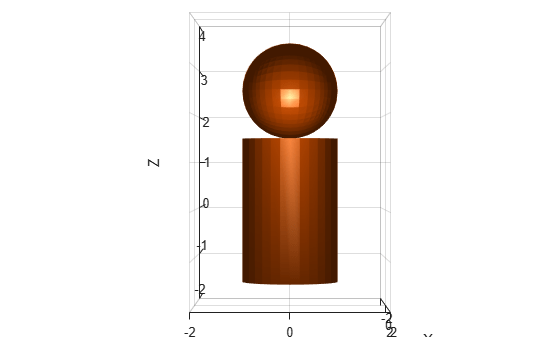# collisionSphere

Create sphere collision geometry

Since R2019b

## Description

Use `collisionSphere` to create a sphere collision geometry centered at the origin.

## Creation

### Syntax

``SPH = collisionSphere(Radius)``
``SPH = collisionSphere(___,Pose=pose)``

### Description

example

````SPH = collisionSphere(Radius)` creates a sphere collision geometry with a specified Radius. The origin of the geometry-fixed frame is at the center of the sphere.```
````SPH = collisionSphere(___,Pose=pose)` sets the `Pose` property of the sphere to `pose`, relative to the world frame.```

## Properties

expand all

Radius of sphere, specified as a positive scalar. Units are in meters.

Data Types: `double`

Pose of the collision geometry relative to the world frame, specified as a 4-by-4 homogeneous matrix or an `se3` object. You can change the pose after you create the collision geometry.

Note

Note that when the pose is specified as an `se3` object, the `Pose` property stores the pose as a numeric 4-by-4 matrix.

Data Types: `single` | `double`

## Object Functions

 `show` Show collision geometry `convertToCollisionMesh` Convert collision primitive geometry into collision mesh geometry `fitCollisionCapsule` Fit collision capsule around collision geometry

## Examples

collapse all

Create a sphere collision geometry centered at the origin. The sphere has a radius of 1 meter.

```rad = 1; sph = collisionSphere(rad)```
```sph = collisionSphere with properties: Radius: 1 Pose: [4x4 double] ```

Visualize the sphere.

```show(sph) title("Sphere")```Create a cylinder collision geometry of radius 1 meter and length 3 meters.

`cyl = collisionCylinder(1,3);`

Create a homogeneous transformation that corresponds to a translation of 2.5 meters up the z-axis. Set the pose of the sphere to the matrix. Show the sphere and the cylinder.

```mat = trvec2tform([0 0 2.5]); sph.Pose = mat; show(sph) hold on show(cyl) view(90,0) zlim([-2 4])```## Version History

Introduced in R2019b

expand all